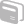# Activities

••• ##### Subject Area

• Math: Algebra I: Functions and Relations

• ##### Author9-12

30 Minutes

• ##### Device
• TI-Navigator™
• ##### Software

LearningCheck™ Creator

## Polynomial Operations

#### Activity Overview

This is a learn check file that includes problems that require the student to 1. Distinguish between the different type of polynomials (monomials, binomials, etc). 2. Add, subtract, and multiply polynomials. 3. Solve geometric word problems that involve adding, subtracting, or multiplying polynomials.

#### Before the Activity

This is a learn check file that includes problems that require the student to 1. Distinguish between the different type of polynomials (monomials, binomials, etc). 2. Add, subtract, and multiply polynomials. 3. Solve geometric word problems that involve adding, subtracting, or multiplying polynomials.

#### During the Activity

Students will take the Learning Check to demonstrate their understanding of the concepts. The teacher should collect the results via the TI Navigator.

#### After the Activity

The teacher will be able to gauge student strengths and weaknesses on these concepts after students have submitted their Learning Check via the TI Navigator.